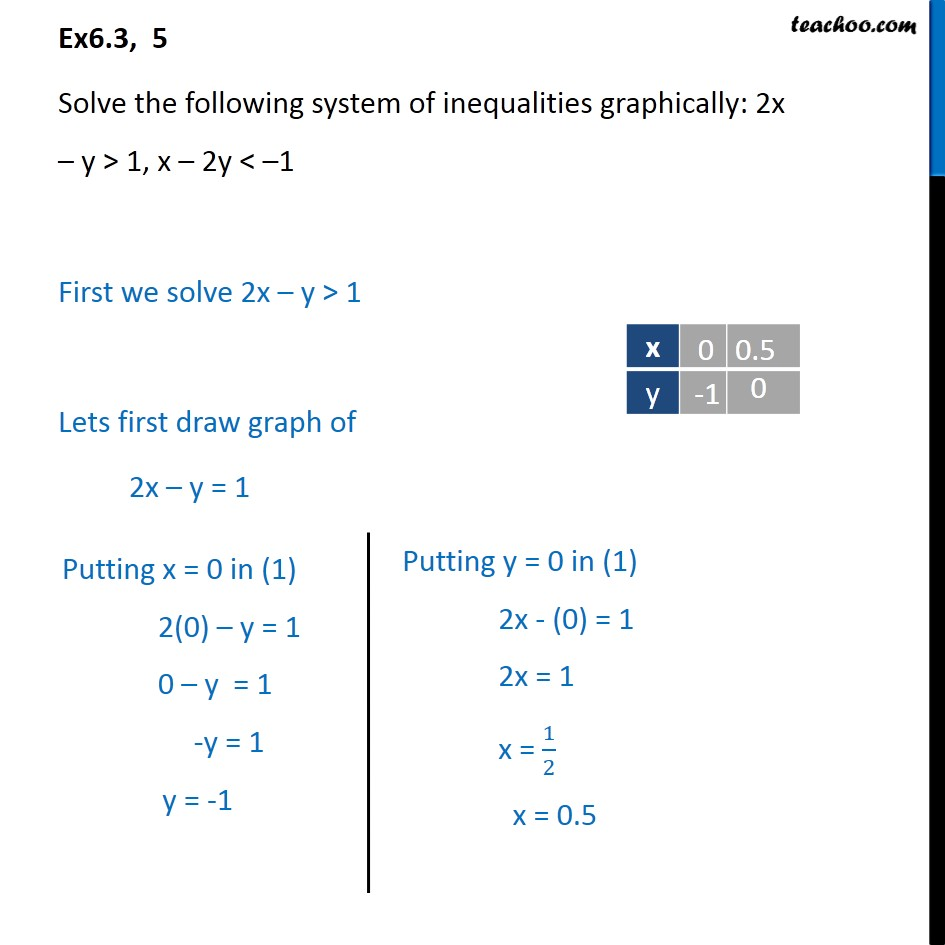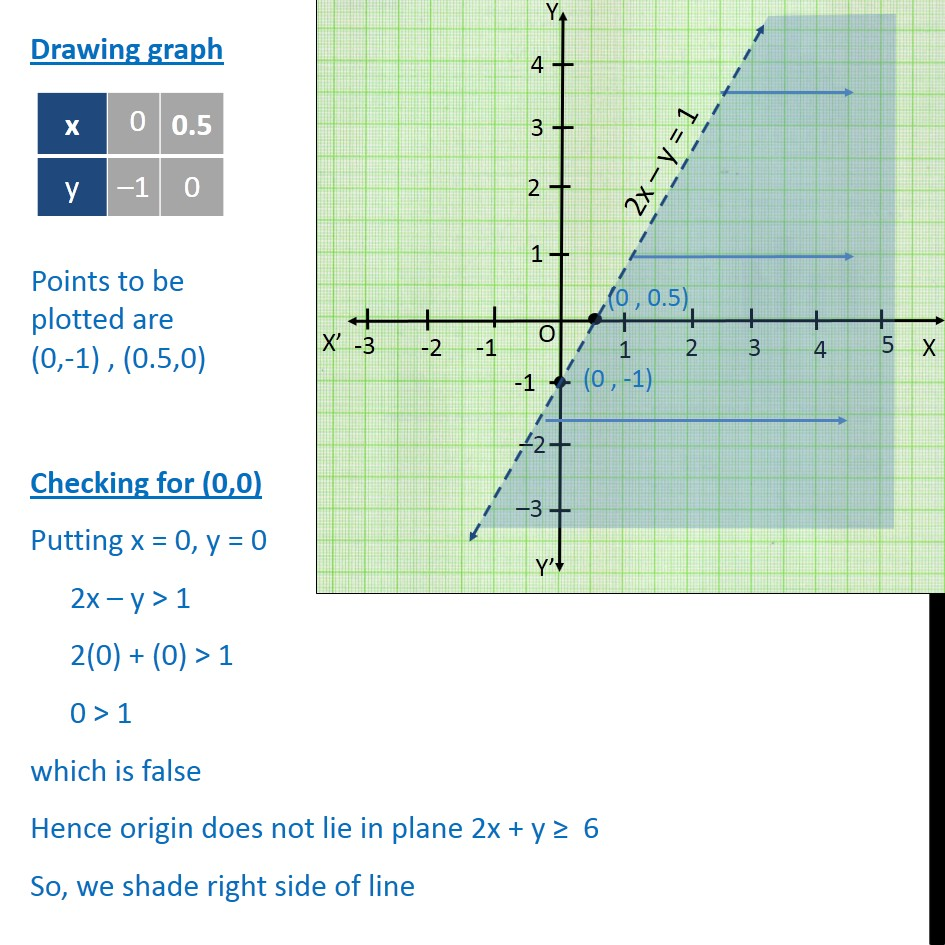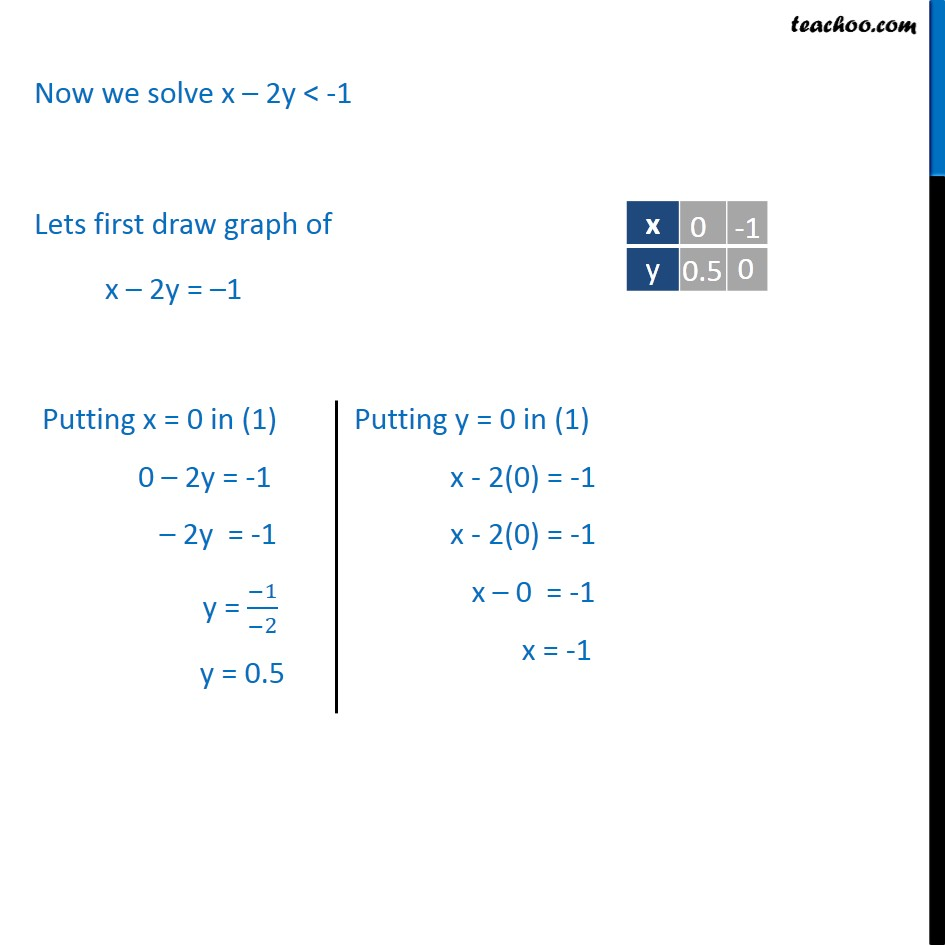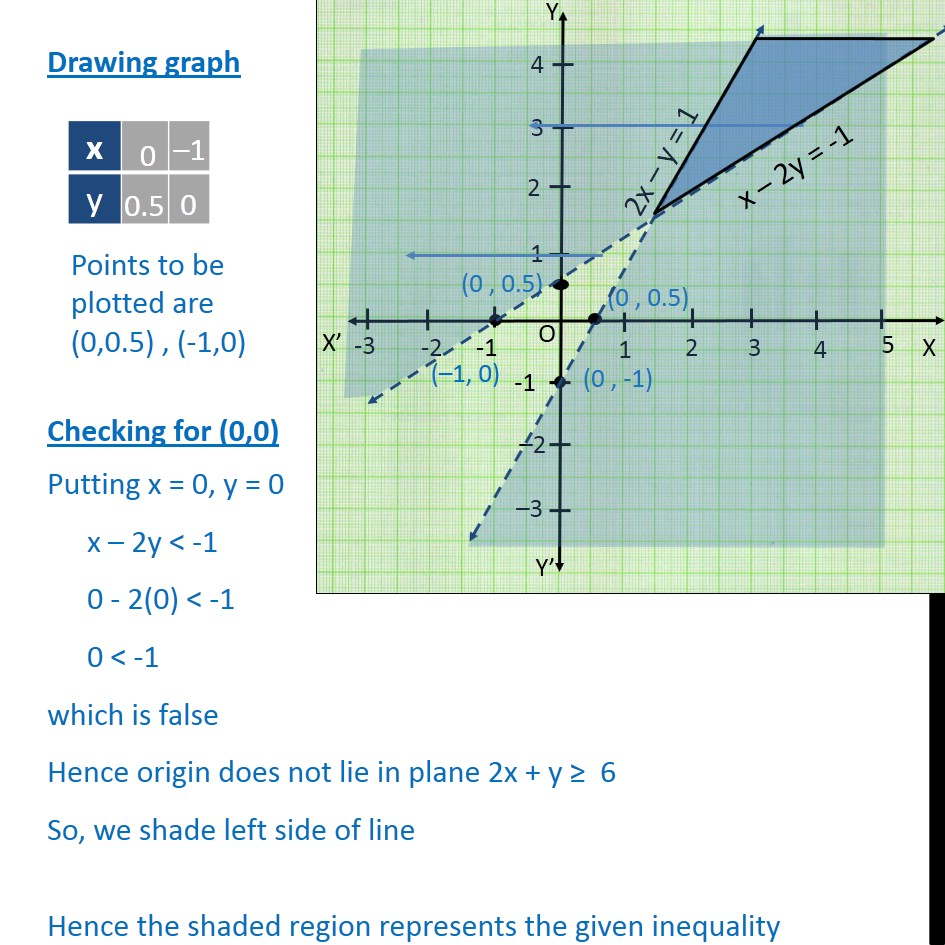Solving Pair of Linear Inequalities

Chapter 5 Class 11 Linear Inequalities
Serial order wiseLearn in your speed, with individual attention - Teachoo Maths 1-on-1 Class

### Transcript

Ex6.3, 5 Solve the following system of inequalities graphically: 2x y > 1, x 2y < 1 First we solve 2x y > 1 Lets first draw graph of 2x y = 1 Drawing graph Checking for (0,0) Putting x = 0, y = 0 2x y > 1 2(0) + (0) > 1 0 > 1 which is false Hence origin does not lie in plane 2x + y 6 So, we shade right side of line Now we solve x 2y < -1 Lets first draw graph of x 2y = 1 Drawing graph Checking for (0,0) Putting x = 0, y = 0 x 2y < -1 0 - 2(0) < -1 0 < -1 which is false Hence origin does not lie in plane 2x + y 6 So, we shade left side of line Hence the shaded region represents the given inequality